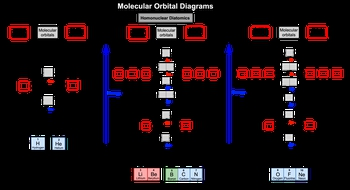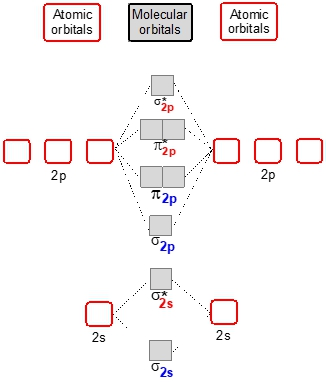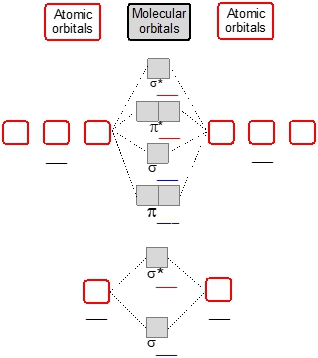## General Chemistry

Learn the toughest concepts covered in Chemistry with step-by-step video tutorials and practice problems by world-class tutors

12. Molecular Shapes & Valence Bond Theory

# MO Theory: Homonuclear Diatomic Molecules

Homonuclear Diatomic Molecules are composed of 2 identical elements bonded together.

Molecular Orbital Diagrams of Homonuclear Diatomics
1
concept

## MO Theory: Homonuclear Diatomic Molecules Concept 13m
Play a video:
Now recall that a whole nuclear diatonic molecule is composed of two identical elements bonded together. Good example of this is n. two or F two. Now as a result of increase in electro negativity and a decrease in atomic size. The order of sigma two P and pi two P. Will be reversed for oxygen to neon. So we take a look here at molecular orbital diagrams in this first one. This correlates to only H two to HE two. So hydrogen and di atomic helium which is not a normal structure. And remember we're looking at the valence electrons for these elements in hydrogen and helium. Their valence electrons are found in the one S orbital for in period one of the periodic table. So here we have for instance this could be the atomic orbital of hydrogen or helium and the other hydrogen or helium. We would fill in the atomic orbital's and then distribute those electrons into our molecular orbital's here. Remember sigma one s. Represents our bonding molecular orbital and sigma star one S. Represents our anti bonding molecular orbital. Now if we move to the next one, this correlates to die atomic lithium all the way to die atomic nitrogen here it gets more complex because now we're dealing with period two elements. So we start off with two S and we move our way up to two P. Now here with the two s orbital is atomic orbital's. We fill in first are bonding molecular orbital which is signaled to us and then start filling in our sigma star to us, which represents our anti body molecular orbital. When we get to two P. Gets more complex as the atomic orbital's continue to pull their electrons together to create new molecular orbital's here. The order for it would be pi two P. Sigma two P. Then we'll go pi star two P. Which is an anti body molecular orbital. Two sigma star two P. Another anti body molecular orbital again. We always fill from lowest molecular orbital up now again because of increasing electro negativity and a decrease in atomic size. We're gonna have a slight change when it comes to die atomic oxygen to die atomic neon. If we look the change happens here they flip. So now my pie toupee moves up And my Sigma two p. moved down. So here again, oxygen to neon there's still period two elements. So we're starting out with two S again and we just start pulling together our atomic orbital electrons and dumping them into our molecular orbital's and start filling it up as we move up. So just keep in mind these are the different types of molecular orbital is that can exist based on what type of period element you're dealing with 12 or three. All right, so keep this in mind as we start doing more and more questions dealing with homo nuclear di atomic molecules2
example

## MO Theory: Homonuclear Diatomic Molecules Example 13m
Play a video:
3
Problem

Determine the number of electrons found in the π2p orbitals for the dioxygen dication, O22+4
Problem

Using a MO diagram, write the electron configuration for the P2 molecule